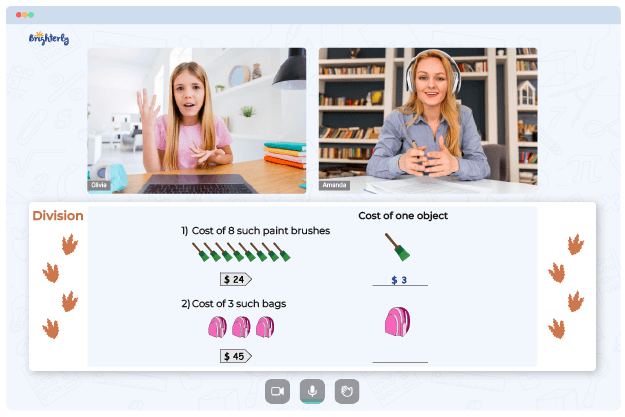Helping students understand long division is essential. Educators often use worksheets to make math learning easier. The division skills introduced in previous grades are revisited and practiced at a more advanced level in the worksheets aimed at fifth graders.

## Why Tutors from Brighterly Use 5th Grade Division Worksheets

Brighterly’s tutors use long division worksheets grade 5 and games to help learners become better at math. Students understand that long division is a technique used in mathematics to break down enormous quantities into more manageable chunks as they use a 5th grade long division worksheet.

Also, students get exposed to the essential terminologies in the long division: dividends, divisors, quotients, and remainders. The dividend is the significant number in a long division problem, while the divisor is the smaller number used to divide it. The quotient represents the numeric value obtained after the division, whereas the remainder describes the undivided portion.

Math for Kids

Is Your Child Struggling With Math?
1:1 Online Math TutoringUsing a long division 5th grade worksheet, kids can learn how to divide, multiply, subtract, bring down, repeat, and then discover the residual or divide again. Similarly, kids will learn how to divide decimal numbers into equal groups using long division.

The quotient generated by dividing a dividend by a divisor represents the total number of possible groupings. At the same time, the remainder indicates the number of components or digits that cannot be grouped.

## How to Use 5th Grade Division Worksheets in a Fun Way### 5th Grade Division Worksheets PDF### 5th Grade Division Worksheets PDF### 5th Grade Division Worksheets PDF### 5th Grade Division Worksheets PDF

When splitting massive numbers, kids will learn how to long divide the problem by breaking it down into smaller, more manageable chunks. They will know that it’s just like conventional division. Students will divide the dividend by the divisor to get the quotient (and often a remainder).

Using 5th grade long division worksheets, kids will understand that long division is a strategy for solving division problems involving huge numbers. It’s the standard approach for resolving division-based difficulties. With time, kids learn how to do the long division in fun and exciting ways using division worksheets grade 5.

### More Division Worksheets

Need help with Division?• Does your child struggle to understand the concept of division?
• Try learning with an online tutor.

Is your child having trouble grasping division concepts? An online tutor could provide the necessary assistance.